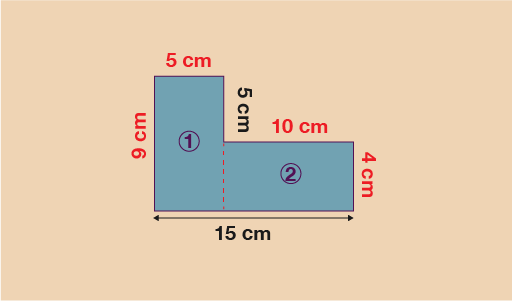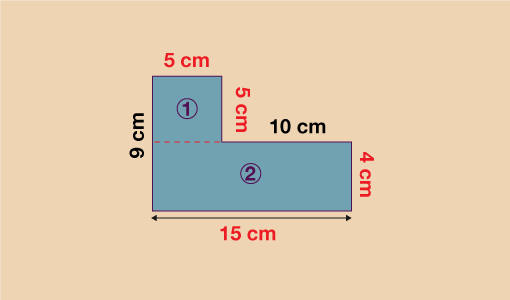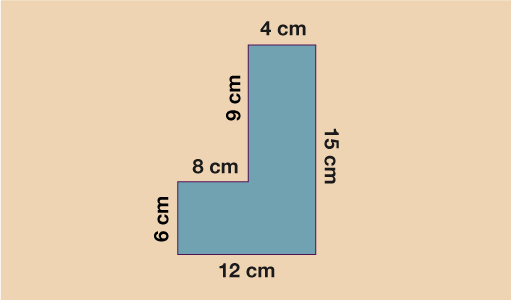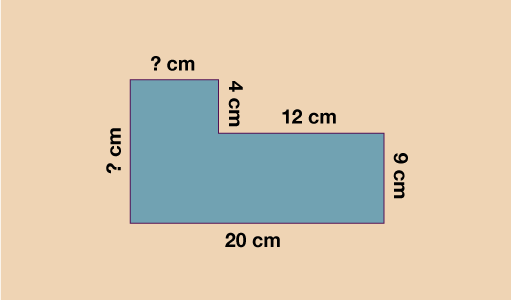Science, Maths & Technology

### Become an OU studentEveryday maths 2

Start this free course now. Just create an account and sign in. Enrol and complete the course for a free statement of participation or digital badge if available.

# 2.2 Area of compound shapes

Take a look at the shape below; this is an example of a compound shape. Whilst you cannot find the area of this shape as it is by using a formula as you have done previously, you can split it into two basic shapes (rectangles) and then use your existing knowledge to work out the area of each of these shapes.Figure 23 Finding the area of a compound shape

You should be able to see that you can split this shape into two rectangles. It does not matter which way you split it (horizontally or vertically), you will get the same answer at the end.

Splitting horizontally:Figure 24 Splitting a compound shape horizontally to find the area

You now have two rectangles. To work out the area of rectangle ①, you do A = 9 × 5 = 45 cm2.

To work out the area of rectangle ②, you do A = 10 × 4 = 40 cm2.

Now that you have the area of both rectangles, simply add them together to find the area of the whole shape:

• 45 + 40 = 85 cm2

You need to be careful that you are using the correct measurements for base and height of each rectangle (the measurements in red). In this example, the lengths of 15 cm and 5 cm (in black) are not required.

If you choose to split the shape vertically:Figure 25 Splitting a compound shape vertically to find the area

Again, you now have two rectangles. To work out the area of rectangle ①, you do A = 5 × 5 = 25 cm2.

To work out the area of rectangle ②, you do A = 15 × 4 = 60 cm2.

Now that you have the area of both rectangles, simply add them together to find the area of the whole shape:

• 25 + 60 = 85 cm2

Again, you need to be careful that you are using the correct measurements for base and height of each rectangle (the measurements in red). In this example, the lengths of 9 cm and 10 cm (in black) are not required.

You will notice that regardless of which way you choose to split the shape, you arrive at the same answer of 85 cm2.

The best way for you to practise this skill is to try a few examples for yourself. Have a go at the activity below and then check your answers.

## Activity 5: Finding the area of compound shapes

Work out the area of the shapes below.

1.Figure 26 Finding the area of compound shapes – Question 1

1. Splitting vertically:
• 6 × 8 = 48 cm2
• 15 × 4 = 60 cm2
• 48 + 60 = 108 cm2
• Splitting horizontally:

• 12 × 6 = 72 cm2
• 9 × 4 = 36 cm2
• 72 + 36 = 108 cm2
1.Figure 27 Finding the area of compound shapes – Question 2

1. The missing lengths are 13 cm (vertical length) and 8 cm (horizontal length).

Splitting vertically:

• 13 × 8 = 104 cm2
• 12 × 9 = 108 cm2
• 104 + 108 = 212 cm2

Splitting horizontally:

• 20 × 9 = 180 cm2
• 4 × 8 = 32 cm2
• 180 + 32 = 212 cm2

Now that you can calculate the area of basic and compound shapes, there is just one last shape you need to be able to find the area of: circles. Similarly to finding the perimeter of a circle, you’ll need to use a formula involving the Greek letter π.